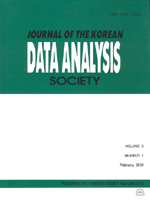상세검색
최근 검색어 전체 삭제
다국어입력
즐겨찾기0KCI등재 학술저널

# Confidence Intervals on Correlation Coefficient in Bivariate Normal Distribution

• 등재여부 : KCI등재
• 2006.08
• 1331 - 1339 (9 pages)

In this paper, we study the confidence intervals of correlation coefficient in a bivariate normal distribution. We find three confidence intervals for the correlation coefficient in a bivariate normal distribution. One is the result of using central limit theorem(CLT), another is that of variance stabilization(VS), and the other is that of Wald type. And we introduce Fisher s confidence interval for the correlation coefficient. Simulation results show that the confidence interval obtained by VS is moderate of four confidence intervals.

1. Introduction

2. Main Results

3. Simulation Results

References